Disclosure: This post may contain affiliate links, meaning when you click the links and make a purchase, we receive a commission.

# How to Calculate/Find Mean and Standard Deviation in Excel

The variance, standard deviation and mean deviation are closely related to each other. In this article, you will get to know how to calculate standard deviation in Excel. You will also see the calculation process of mean deviation also.

In statistics, standard deviation calculates a group of data by which you measure how the calculation is spread out from an expected or average value. It is defined as the square root of the dispersion or variance in a frequency distribution. The mean deviation is another measure of dispersion which is defined as the average absolute values of the differences between individual numbers and their arithmetic mean or median.

## Calculate Mean Deviation in Excel with Formula

The mean deviation can be calculated as the mean deviation from mean or mean deviation from the median. If in your calculation the arithmetic mean is subtracted from the individual values then it is called the mean deviation from the mean. If the subtracted item is median then it is called the mean deviation from the median. The formulas for calculating the mean deviation are given below.

Mean Deviation from Mean,where,

• X is each observation
• μ is the arithmetic mean
• N is the total number of observations

Mean Deviation from Median,Where,

• X is each observation
• M is the Median of the observations
• N is the total number of observations

The following picture shows how the mean deviation is calculated in Excel.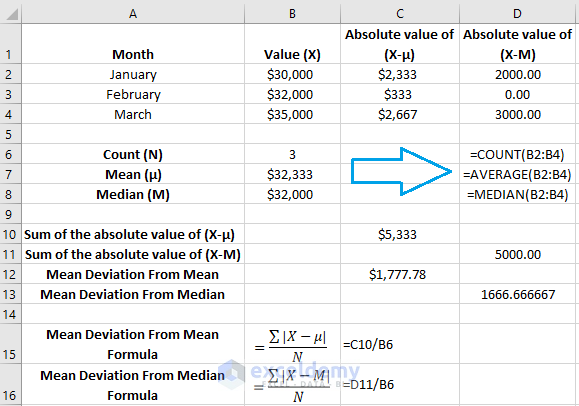In the above example, I took three values for three months. The total number of observations was calculated using the formula =COUNT (B2: B4). For the mean and median calculation, I used the formula =AVERAGE (B2: B4) and =MEDIAN (B2: B4) respectively. To calculate the absolute value of (X-μ) I used the formula =ABS(B2-\$B\$7) in cell C2 and drag this formulated cell to C3 and C4 for copying the formula for the whole column. The same thing was done for calculating the absolute value of (X-M). In cell D2, the formula, =ABS(B2-\$B\$8) was used and it was dragged down for the rest of the cell of column D for continuing the same calculation. After this, the sum of the absolute value of mean (X-μ) and median (X-M) was calculated using the formula =SUM (C2: C4) and =SUM (D2: D4) in cell C10 and D11 respectively. These all are the necessary values that are needed for calculating the mean deviation. Now for calculating the mean deviation from the mean I used the formula =C10/B6 in cell C15 and for calculating the mean deviation from the median I used the formula =D11/B6 in cell C16. This is how the whole calculation was done for calculating the mean deviation.

## How to Calculate Standard Deviation In Excel with Formula

To calculate the standard deviation, you need to calculate the variance first as the standard deviation is the square root of the variance. The standard deviation can be of two kinds. They are population standard deviation and sample standard deviation. The formula for calculating the standard deviation is given below.

Population Standard Deviation,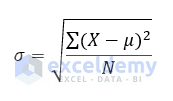Sample Standard Deviation,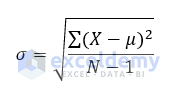Here for both the equations,

• μ is the arithmetic mean
• X is the individual value
• N is the size of the population
•  is the standard deviation

To calculate the standard deviation along with the variance the prime requirement is to calculate the deviation about the mean. Deviation about the mean is calculated by subtracting the arithmetic mean with each individual value. The formula for calculating the arithmetic mean is =AVERAGE (B2: B6). I applied this formula in cell B11. To get the value of deviation about the mean (X-μ) apply the formula =B2-\$B\$11 in cell C2 and drag this formula downwards to apply it for the whole column. Write the formula =C2^2 in cell D2 and drag it downwards to copy the formula for the whole column to find the square of the deviation about the mean. Now to find the sum of the square of the deviation about the mean apply the formula =SUM (D2: D6) in cell B9. For counting the total number of data (N) write =COUNT (B2: B6) in cell B10. Now, apply the formula B9/B10 and B9/(B10-1) in cell B12 and B14 respectively to calculate the population and sample variance. Finally, you need to find the square root of the variance to perform the standard deviation. Here, to find the standard deviation in cell B13 and B15 I perform the square root using the formula =B12^0.5 and =B14^0.5 respectively.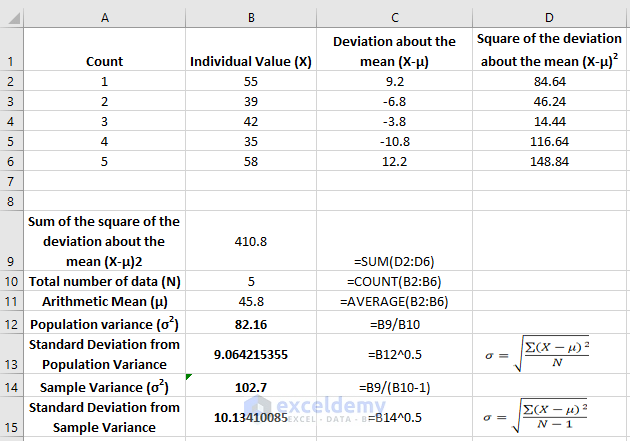Read More: How to calculate Average, Median, & Mode in Excel

## Calculating the Mean & Standard Deviation Using the Built-in Excel Functions

In excel there is a built-in function to calculate the mean deviation from the mean. But for the mean deviation from the median, there is no built-in function. For calculating the standard deviation there are 6 functions in excel. The following picture shows the result of mean deviation from the mean, population standard deviation and sample standard deviation using the built-n excel functions.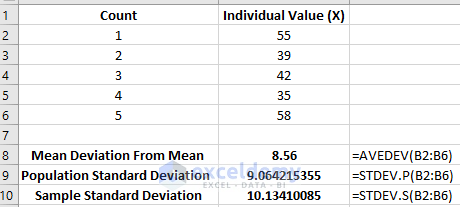Here, for calculating the mean Deviation From mean I used function AVEDEV. The formula =AVEDEV (B2: B6) is applied in cell B8. The functions for calculating the standard deviation for an entire population is STDEV.P and the function for calculating the standard deviation from a sample of a population is STDEV.S. These two functions ignore the text and logical values. If you want to evaluate the logical/text value in your calculation you can use the functions STDEVPA and STDEVA. STDEVPA calculates the population standard deviation and STDEVA calculates the sample standard deviation by evaluating the logical or text values. In the below picture I used the available 4 functions for calculating the standard deviation. As there is a text value present in the observation, the result is different in every case.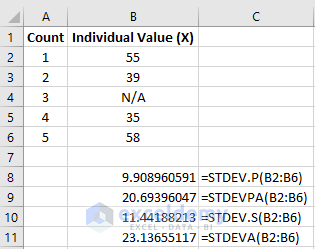The other two functions available for calculating the standard deviation are STDEV and STDEVP. Both these functions are compatible with Excel 2007 and its earlier versions.

## Conclusion

Standard and mean deviations are the basics of statistical analysis. In any kind of business modeling, they play a huge role. You can not compare your data without knowing the deviations.

In this article, I tried to explain and calculate the standard and mean deviations by using different built-in excel functions and formula. While performing the formulas I had to calculate many other factors which took a lot of time. Excel ignores this factors by simply using the built-in functions.

The excel file where all these calculations were done is given below.